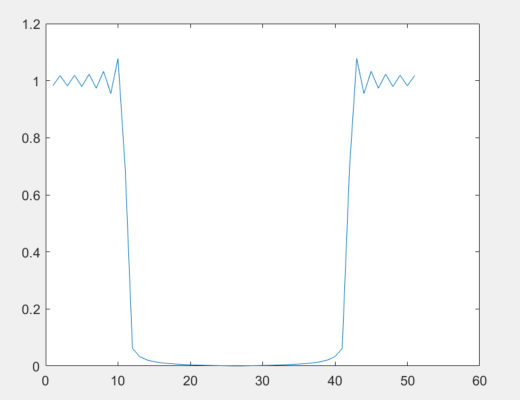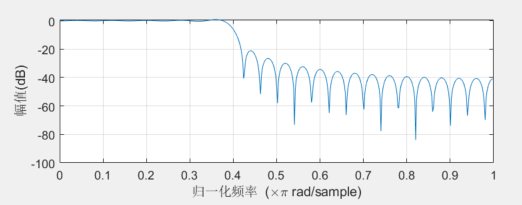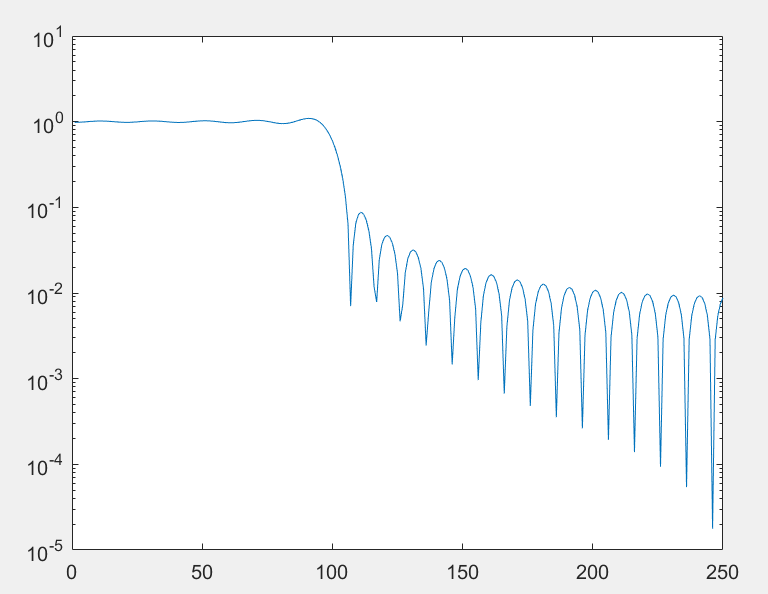# 问题

``````b = 0.4*sinc(0.4*(-25:25));
plot(abs(fft(b)));````````````b = 0.4*sinc(0.4*(-25:25));
freqz(b);``````# 调查一下

``````b = 0.4 * sinc(0.4 * (-25 : 25));
res = abs(fft(b, 500));
semilogy(res(1 : length(res) / 2));
``````